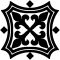#Blackmoor Vituperative

## Sunday, 2021-04-18

### Rounding to significant digits in LibreOffice Calc

Filed under: Software — bblackmoor @ 12:24

Here is a formula for rounding a number to a specified number of significant digits.

`=if(A1=0,0,round(10^(floor(B1-LOG(abs(A1))))*A1)/(10^(FLOOR(B1-log(abs(A1))))))`

In this formula, A1 is the cell with the number, and B1 is the cell with the number of significant digits.